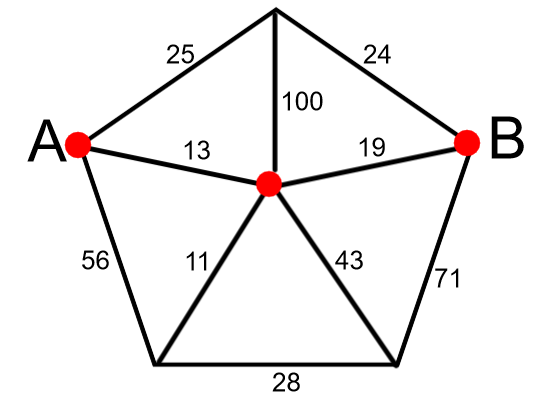# Friday Challenge: 17th JulyLook at this diagram.How many different routes can you find from A to B?

Calculate the value of each route.For example: 13 + 43 + 71 = 127

What is the route with the lowest value?

What is the route with the highest value?

EXTENSION: Can you design a diagram with slightly more challenging arithmetic problems?